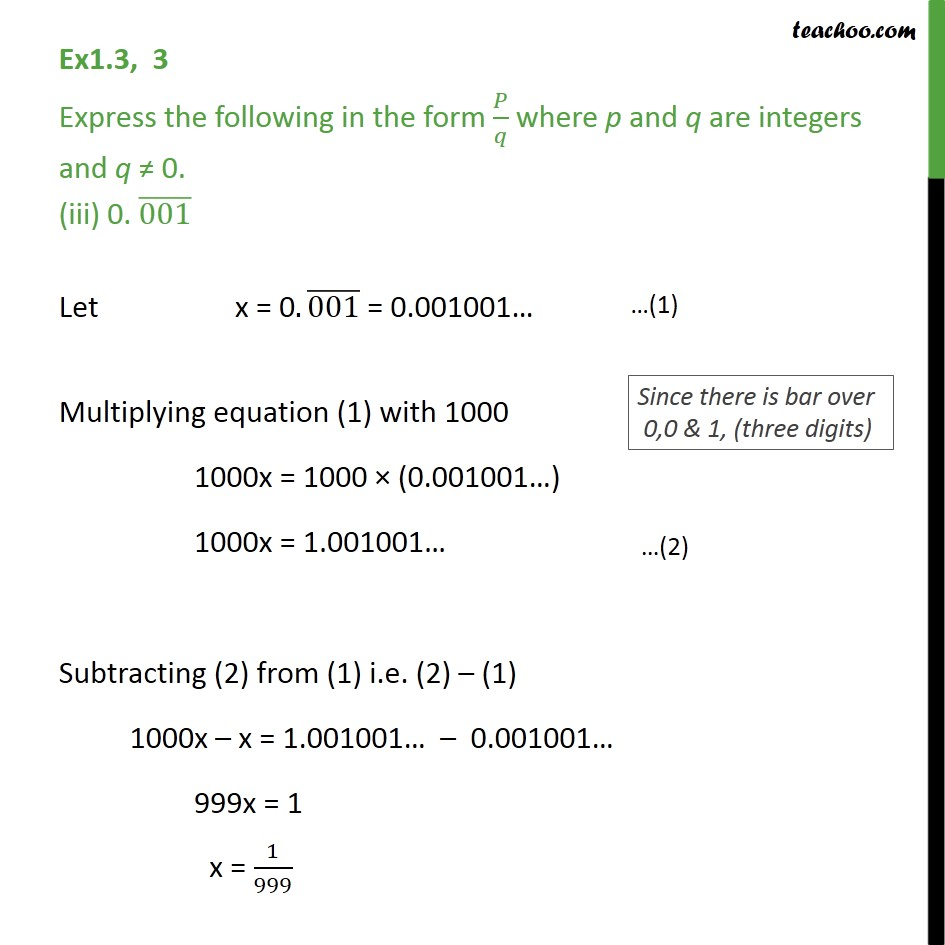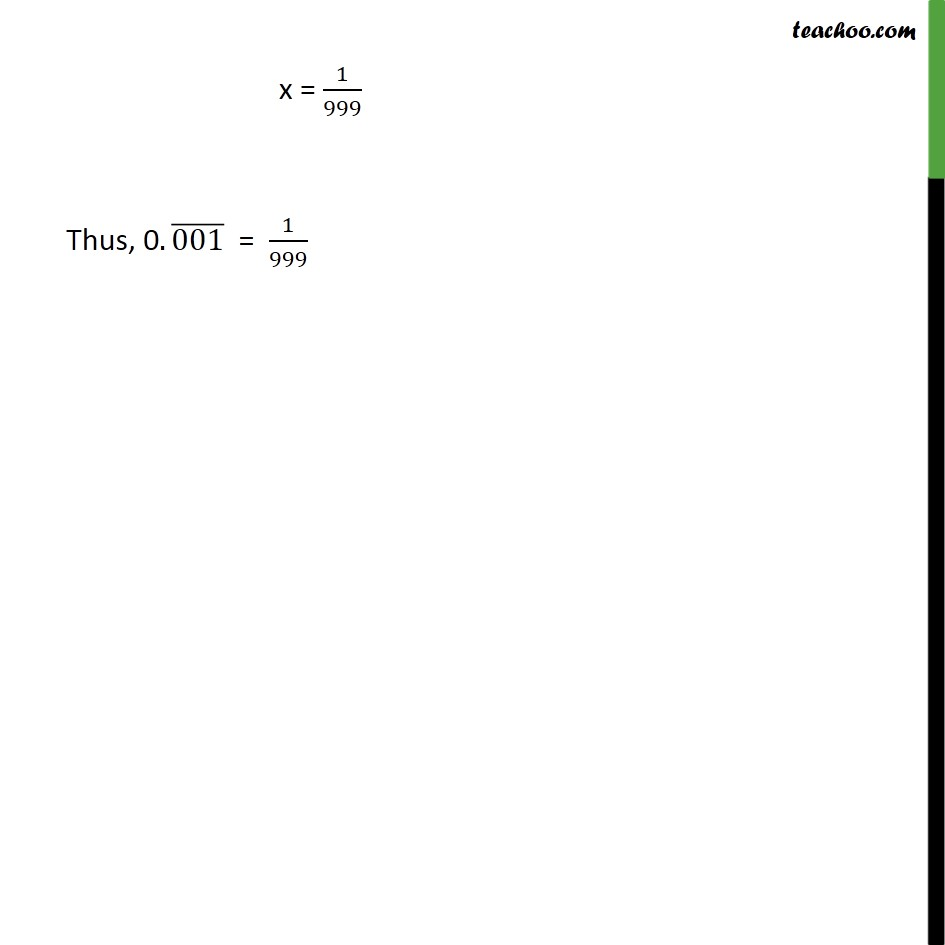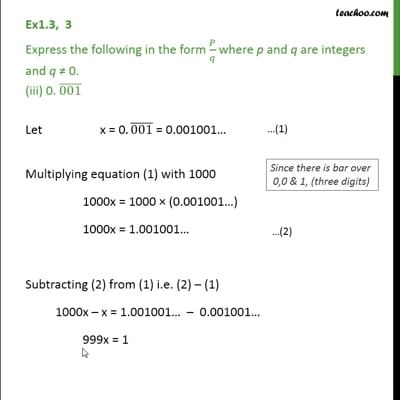Ex 1.3

Chapter 1 Class 9 Number Systems (Term 1)
Serial order wiseThis video is only available for Teachoo black users

### Transcript

Ex1.3, 3 Express the following in the form 𝑃/𝑞 where p and q are integers and q ≠ 0. (iii) 0. (001) ̅ Let x = 0.(001) ̅ = 0.001001… Multiplying equation (1) with 1000 1000x = 1000 × (0.001001…) 1000x = 1.001001… Subtracting (2) from (1) i.e. (2) – (1) 1000x – x = 1.001001… – 0.001001… 999x = 1 x = 1/999 x = 1/999 Thus, 0.(001) ̅ = 1/999